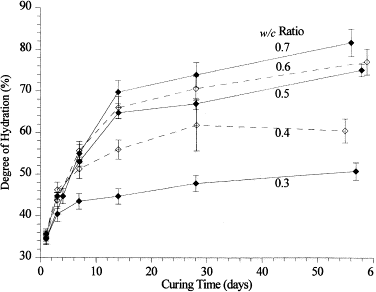Kinetics of cement: Degree of hydration or Degree of reaction?

It is so common to see the terms “degree of hydration” and “degree of reaction” in research field of cemetitious material, such as cement or blended cement, especially when we focus on the kinetics.

Here comes the question, what is the difference between the two terms? are they actually the same meaning? I was confused by the two terms when I read a huge number of papers and found not so many researchers make them clear or maybe they do not realize the difference either.Degree of hydration, α, as a function of curing time (By Raymond A. Cook).

First, let’s know what cement hydration is? it means chemical combination of cement and water. Then what is hydration degree of cement? “Degree of hydration” of cement is defined as the fraction of Portland clinker (including gypsum addition) that has fully reacted with water.

There is no need to explain the meaning of word “reaction”. However, “Degree of reaction” of cement is defined as the fraction of Portland clinker (including gypsum addition) that has already fully reacted with water relative to the final reacted cement.

From the two definitions for the two terms, the difference is clear. Degree of hydration does not consider the fact that ultimate hydration, i.e. hydration degree is 100%, does not occur in reality. While degree of reaction is defined as the reacted part relative to the whole part that will be finally reacted, in other words, 100% reaction degree is possible.

Because complete hydration of cement does not happen, ultimate hydration degree of cement is introduced. For Portland cement the ultimate degree of hydration can be calculated using Mill’s formula. Reference value of ultimate hydration degree of Portland cement (water-to-cement ratio ≈ 0.5) could be 75%, yes, the corresponding reaction degree is 100%.